# Analysis of Half-life Regression Model Made by Duolingo

Last year Burr Settles (from Duolingo) and Brendan Meeder proposed a model predicting the probability of recall during the practice with Duolingo based on the lag time since a particular item was last presented by a learner. The original paper is called A Trainable Spaced Repetition Model for Language Learning (video) and the idea is also described in this blog post.

Since I am a Ph.D. candidate in Adaptive Learning Group and my research is focused on computerized adaptive practice of factual knowledge and issues related to its evaluation, I am very interested in papers produced by Duolingo employees (e.g., I recommend a paper Mixture Modeling of Individual Learning Curves). I presented the paper at our seminar on March 22 and thanks to the fact that authors published not only the paper, but also the source code of the model and data used for its evaluation, I was able to analyze behavior of the model and its evaluation more closely.

To understand the analysis which follows, please read the original paper or blog post. The full code of this analysis is available on GitHub and is based on the fork of the original repository to minimize the probability of my error.

If you want to this Jupyter notebook on your own, please download the original data set to the data directory and run the experiment:

pypy experiment.py -m hlr ./data/settles.acl16.learning_traces.13m.csv.gz

After you run the command above, you should see something like this in your console:

method = "hlr"
reading data...0...1000000...2000000...3000000...4000000...5000000...6000000...
7000000...8000000...9000000...10000000...11000000...12000000...done!
|train| = 11568803
|test|  = 1285423
test    191801294.6 (p=109014.3, h=191692154.1, l2=126.2)   mae(p)=0.129
cor(p)=0.038    mae(h)=117.454  cor(h)=0.203
In :
%matplotlib inline
%load_ext autoreload
%autoreload 2

The autoreload extension is already loaded. To reload it, use:
%reload_ext autoreload

In :
from evaluation import plot_model_stats
from models import train_test_set, ItemAverage
import matplotlib.pylab as plt
import numpy as np
import pandas as pd
import seaborn as sns

sns.set(style="white")

DAY_SECONDS = 60 * 60 * 24


## Analysis of the Input Traces¶

Since the paper is about finding the optimal learner model handling forgetting, we start with the analysis of available data, because the data usually matters a lot, see Impact of Data Collection on Interpretation and Evaluation of Student Models.

In :
traces = pd.read_csv('./data/settles.acl16.learning_traces.13m.csv.gz')

In :
traces['delta_days'] = traces['delta'].apply(lambda d: d / DAY_SECONDS)

In :
traces.head()

Out:
p_recall timestamp delta user_id learning_language ui_language lexeme_id lexeme_string history_seen history_correct session_seen session_correct delta_days
0 1.0 1362076081 27649635 u:FO de en 76390c1350a8dac31186187e2fe1e178 lernt/lernen 6 4 2 2 320.018924
1 0.5 1362076081 27649635 u:FO de en 7dfd7086f3671685e2cf1c1da72796d7 die/die 4 4 2 1 320.018924
2 1.0 1362076081 27649635 u:FO de en 35a54c25a2cda8127343f6a82e6f6b7d mann/mann 5 4 1 1 320.018924
3 0.5 1362076081 27649635 u:FO de en 0cf63ffe3dda158bc3dbd55682b355ae frau/frau 6 5 2 1 320.018924
4 1.0 1362076081 27649635 u:FO de en 84920990d78044db53c1b012f5bf9ab5 das/das 4 4 1 1 320.018924

### Observed Recall Rate¶

In :
plt.hist(traces['p_recall'], bins=50)
plt.ylabel('Number of iteractions')
plt.xlabel('Probability of recall')
plt.show()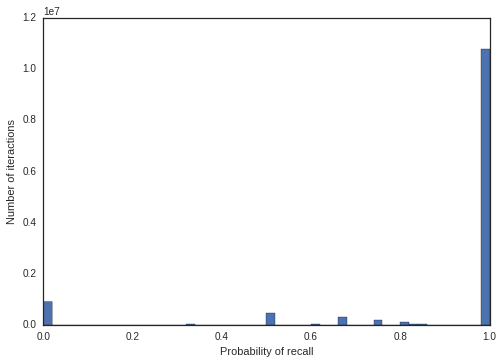The most of interactions leads to high probability of recall. There is a danger that the proposed model will ignore some interactions to achieve higher accuracy

### Distribution of Lag Times¶

In :
plt.hist([x for x in traces['delta_days'] if x < 365], bins=20)
plt.xlabel('Days')
plt.ylabel('Number of answers')
plt.yscale('log')
plt.show()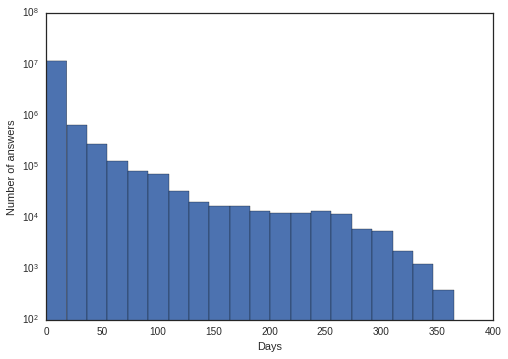You can see that the most of the interactions have only small lag time, so the fitted model will probably focus mainly on the interactions having lag time lower than 10 days.

### Length of Traces¶

In :
plt.hist([
x for x in traces.sort_values(by=['timestamp']).drop_duplicates(['user_id', 'lexeme_id'])['history_seen']
if x <= 20
], bins=20)
plt.xlim(1, 20)
plt.xlabel('Length')
plt.ylabel('Number of series')
plt.show()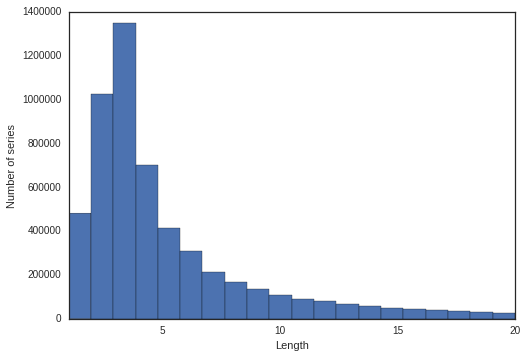The most of series (a learner's interactions for one item) has a length of three, or two.

### Forgetting¶

In :
def _compute_bin_p_recall(group):
return pd.DataFrame([
{'p_recall': group['p_recall'].mean()}
])
global_forgetting = traces.groupby(
pd.qcut(traces['delta_days'], 20)
).apply(_compute_bin_p_recall).reset_index().drop(['level_1'], axis=1)
sns.barplot(x='delta_days', y='p_recall', data=global_forgetting, color=sns.color_palette())
plt.xticks(rotation=90)
plt.ylim(0.85, 0.95)
plt.show()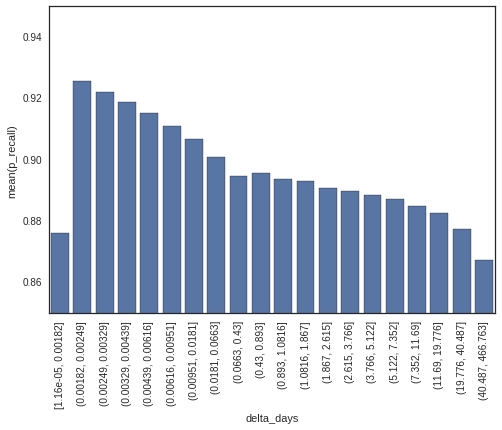Suprisingly, the probability of recall does not only decrease with higher lag time. An average probability of the recall is the lowest in the case of really low lag time. Probably, in this case lexemes are not fully learned yet. Unfortunately, this phenomenon is not handled by the proposed model by design.

## Analysis of the Results¶

The paper presents the following features used in half-life regression:

1. interaction features
• number of all correct answers (square root)
• number of all wrong answers (square root)
2. lexeme tag features
• bias dependent on a lexeme

I really do not underestand why there is no learners' feature (e.g., UI language, or estimated prior skill).

In :
results = pd.read_csv('./results/hlr.settles.acl16.learning_traces.13m.preds', delimiter='\t')

In :
results.head()

Out:
p pp h hh lang user_id timestamp
0 0.9999 0.8741 274 168.7282 en->fr u:dHVF 1363009539
1 0.9999 0.9136 274 175.2046 en->fr u:dHVF 1363009539
2 0.9999 0.8803 274 124.2595 en->fr u:dHVF 1363009539
3 0.9999 0.8969 274 145.5307 en->fr u:dHVF 1363009539
4 0.9999 0.9005 274 165.1536 en->fr u:dHVF 1363009539

### Calibration¶

In :
plot_model_stats(results['pp'], results['p'], bins=50)

RMSE: 0.2912178924698432
MAE: 0.128756880575
AUC: 0.537534701083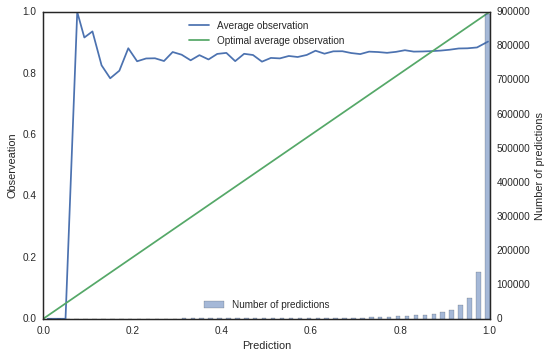Using MAE for the evalution of a learner model is questionable, see Metrics for Evaluation of Student Models paper for more information. The final model is really bad in the predictive accuracy (ideally, the blue line should be aligned with the green one). It seems that the presented model has almost no predictive power. Also the diversity of predictions is really low, almost all predictions are near to one.

### Comparison to Item Average¶

Imagine a learner model which ignores learning and forgetting. This model just computes an average probability of recall per item and uses it as a prediction for the future interactions.

It is worth noting that train/test division is not the same as the one used to fit and evaluate half-life regression model. However, I assume it does not affect a general messsage of the analysis.

In :
trainset, testset = train_test_set(traces)

In :
item_average = ItemAverage()
item_average.train(trainset)

In :
predicted = np.zeros(len(testset))
for i, lexeme_id in enumerate(testset['lexeme_id'].values):
predicted[i] = item_average.predict(lexeme_id)
plot_model_stats(predicted, testset['p_recall'], 50)

RMSE: 0.26902643250089264
MAE: 0.170920653476
AUC: 0.606297726705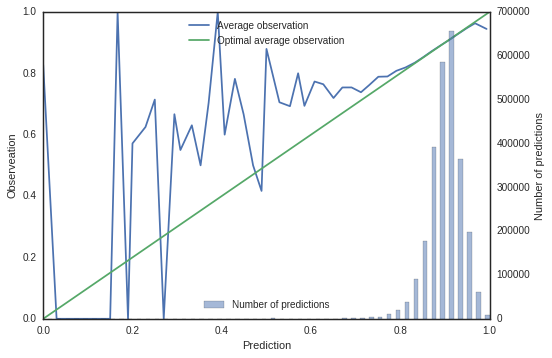You can see that even this simple model is much better than the one presented in the original paper. I understand that the goal of the paper is to find a parameter controlling degradation of skill meters and good predictive accuracy is a bonus. However, I assume that if there is a model ignoring learning and forgetting better than the proposed one, there is also a much better model taking learning and fogetting into accout.